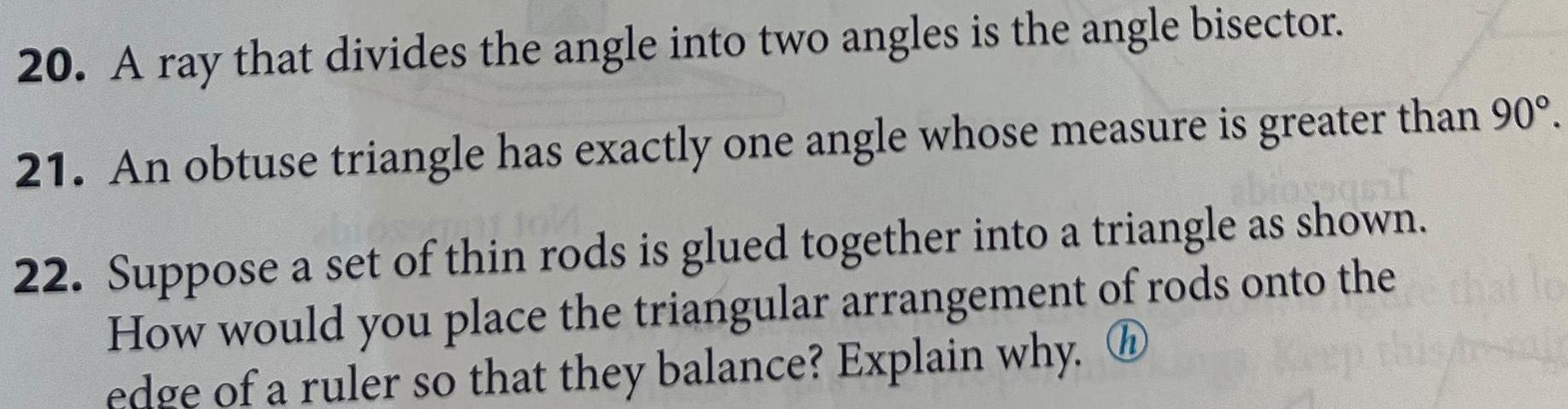Question:

# 20 A ray that divides the angle into two angles is the angle

Last updated: 9/15/202320 A ray that divides the angle into two angles is the angle bisector 21 An obtuse triangle has exactly one angle whose measure is greater than 90 biosoqsil 22 Suppose a set of thin rods is glued together into a triangle as shown How would you place the triangular arrangement of rods onto the edge of a ruler so that they balance Explain why